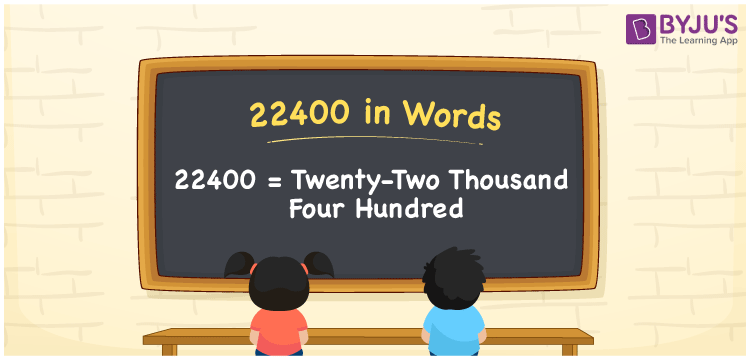# 22400 in Words

22400 in words is written as “Twenty-Two Thousand Four Hundred”. For example, there are Twenty-Two Thousand Four Hundred gallons of water in a tank. In Mathematics, 22400 is a cardinal number, that represents the quantity or value of something. Let us see in this article how to write 22400 in words using the place value chart.

 22400 in Words Twenty-Two Thousand Four Hundred Twenty-Two Thousand Four Hundred in Numbers 22400

## 22400 in English Words## How to Write 22400 in Words?

22400 is a five-digit number that can be written in words, by knowing the place value of each digit in the number. This can be done using a place value chart.

 Ten  Thousands Thousands Hundreds Tens Ones 2 2 4 0 0

We can see, from the above table, the positions of digits are:

• 2 → Ten Thousands
• 2 → Thousands
• 4 → Hundreds
• 0 → Tens
• 0 → Ones

Hence, when we read the number from right to left, it is Twenty-Two Thousand Four Hundred. Learn more about numbers in words at BYJU’S.

### Expanded Form of 22400

We can write the expanded form as:

2 × Ten thousand + 2 × Thousand + 4 × Hundred + 0 × Ten + 0 × One

= 2 × 10000 + 2 × 1000 + 4 × 100 + 0 × 10 + 0 × 1

= 20000 + 2000 + 400 + 0 + 0

= 22400

= Twenty-Two Thousand Four Hundred

22400 is the natural number that is succeeded by 22399 and preceded by 22401. Learn more about the number 22400 below:

• 22400 in Words – Twenty-Two Thousand Four Hundred
• Is 22400 an odd number? – No
• Is 22400 an even number? – Yes
• Is 22400 a perfect square number? – No
• Is 22400 a perfect cube number? – No
• Is 22400 a prime number? – No
• Is 22400 a composite number? – Yes

## Frequently Asked Questions on 22400 in words

Q1

### What is 22400 in words?

22400 in words is expressed as Twenty-Two Thousand Four Hundred.
Q2

### What is the place value of 4 in 22400?

The place value of 4 in 22400 is Hundreds.
Q3

### How to write Rs.22400 in a cheque ?

Rs.22400 is written as Twenty-Two Thousand Four Hundred only in a cheque.